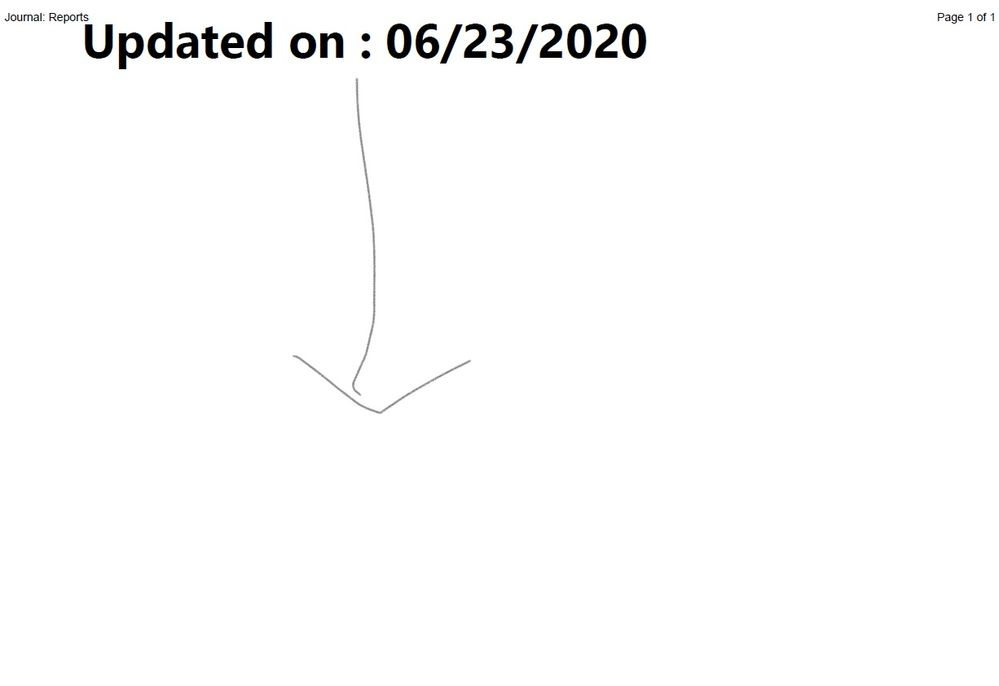Choose Language Hide Translation Bar
Highlighted

## Display Text at center of the Page in Journal

How do i able to display Text at center of the page in Journal?

``````Names Default To Here( 1 );
Clear Symbols();
Clear Log();
Close All( Data Tables, NoSave );
Close All( Journals, NoSave );

nw = New Window( "Reports",
<<Journal,
hlb = H List Box(
lub = Lineup Box( N Col( 1 ) )
)
);

lub << Append( tb = Text Box( "Updated on : " || Short Date( Today() ) ));
tb << Set Font Size( 50 ) << Set Font Style( "Bold" ) << Justify Text( "Center" );
tb << set width(1000) << set wrap(1000);

Reports = Current Journal();
Reports << Set page setup( margins( 0.1, 0.1, 0.1, 0.1 ), scale( 0.8 ), portrait( 0 ), paper size( "A4" ) );

Reports << get page setup();
Reports << SavePDF("DSA_report.pdf");``````

The layout that i intended like picture below.1 ACCEPTED SOLUTION

Accepted Solutions
Highlighted

## Re: Display Text at center of the Page in Journal

``````//With colors for better understanding
nw = New Window( "Reports",
<<Journal,
hlb = H List Box( Spacer Box(<<Color(red)), V List Box( Spacer Box(<<Color(blue)), hlb2 = H Center Box(), Spacer Box(<<Color(green)) ), Spacer Box(<<Color(yellow)) )
);

hlb2 << Append( tb = Text Box( "Updated on : " || Short Date( Today() ) ) );
tb << Set Font Size( 10 ) << Set Font Style( "Bold" );

//Without colors
nw = New Window( "Reports",
<<Journal,
hlb = H List Box( Spacer Box(), V List Box( Spacer Box(), hlb2 = H Center Box(), Spacer Box() ), Spacer Box() )
);

hlb2 << Append( tb = Text Box( "Updated on : " || Short Date( Today() ) ) );
tb << Set Font Size( 10 ) << Set Font Style( "Bold" );``````
2 REPLIES 2
Highlighted

## Re: Display Text at center of the Page in Journal

``````//With colors for better understanding
nw = New Window( "Reports",
<<Journal,
hlb = H List Box( Spacer Box(<<Color(red)), V List Box( Spacer Box(<<Color(blue)), hlb2 = H Center Box(), Spacer Box(<<Color(green)) ), Spacer Box(<<Color(yellow)) )
);

hlb2 << Append( tb = Text Box( "Updated on : " || Short Date( Today() ) ) );
tb << Set Font Size( 10 ) << Set Font Style( "Bold" );

//Without colors
nw = New Window( "Reports",
<<Journal,
hlb = H List Box( Spacer Box(), V List Box( Spacer Box(), hlb2 = H Center Box(), Spacer Box() ), Spacer Box() )
);

hlb2 << Append( tb = Text Box( "Updated on : " || Short Date( Today() ) ) );
tb << Set Font Size( 10 ) << Set Font Style( "Bold" );``````
Highlighted

## Re: Display Text at center of the Page in Journal

So, we can make use of the spacer box and adjust it manually. I thought there are other way to do it. Thanks for input.

Article Labels